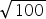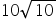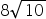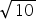Mathematics
Easy

Question

# Rewriteinto simplest form

## 10Hint:

## The correct answer is: 10

### Here, we have to write the simplest form of √100 .Now, √100 = √ ( 2 × 2 × 5 × 5 ) .= √ ( 22 × 52 )= (2 × 5 )= 10Hence, the correct option is (a).

The square root is an inverse method of squaring of a number.

### Related Questions to study#### With Turito Foundation.#### Get an Expert Advice From Turito.